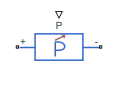Dynamic load for DC or AC supply

Since R2020b

•Libraries:
Simscape / Electrical / Passive

## Description

The Dynamic Load block implements a dynamic load for a DC or AC supply.

If you set the Load type parameter to `DC`:

• The consumed power of the block is equal to the P port as long as it is greater than the value of the Minimum consumed power parameter and the voltage from the DC supply is equal to or greater than the value specified for the Minimum supply voltage parameter.

• When the voltage from the DC supply drops below the Minimum supply voltage, the load behaviour changes and the block acts as a resistive load. If the supply voltage becomes negative, the block acts as an open circuit conductance.

To ensure smooth transitions between these behaviours, the block uses a third-order polynomial spline with continuous derivatives. You can specify the width of this transition using the Transition voltage width parameter.

If you set the Load type parameter to `AC`, this table shows the relationship between the voltage from the AC supply, V, the active power at the P port, Pin, and the consumed active power of the block, Pactual:

Applicable Range of V ValuesApplicable Range of Pin ValuesCorresponding Consumed Active Power
$V>{V}_{\mathrm{min}}$${P}_{in}>{P}_{\mathrm{min}}$$\begin{array}{l}{P}_{actual}={P}_{in}\\ {Q}_{actual}={Q}_{in}\end{array}$
${P}_{in}<{P}_{\mathrm{min}}$${P}_{actual}={P}_{\mathrm{min}}$
$V<{V}_{\mathrm{min}}$${P}_{in}>{P}_{\mathrm{min}}$The block models a load with an impedance equal to:$\begin{array}{l}R={V}_{\mathrm{min}}^{2}\frac{{P}_{in}}{{P}_{in}^{2}+{Q}_{in}^{2}}\\ X={V}_{\mathrm{min}}^{2}\frac{{Q}_{in}}{{P}_{in}^{2}+{Q}_{in}^{2}}\\ Z=\sqrt{{R}^{2}+{X}^{2}}\end{array}$
${P}_{in}<{P}_{\mathrm{min}}$${P}_{actual}={P}_{\mathrm{min}}$

Where:

• Vmin is the value of the Minimum supply voltage (RMS) parameter.

• Pmin is the value of the Minimum active power parameter.

• Pactual is the consumed active power of the Dynamic Load block.

• Qactual is the consumed reactive power of the Dynamic Load block.

The consumed reactive power of the block is equal to the Q port as long as the voltage from the AC supply is equal to or greater than the value specified for the Minimum supply voltage (RMS) parameter. Otherwise, the block models a load with an impedance equal to:

`$\begin{array}{l}R={V}_{\mathrm{min}}^{2}\frac{{P}_{in}}{{P}_{in}^{2}+{Q}_{in}^{2}}\\ X={V}_{\mathrm{min}}^{2}\frac{{Q}_{in}}{{P}_{in}^{2}+{Q}_{in}^{2}}\\ Z=\sqrt{{R}^{2}+{X}^{2}}\end{array}$`

.

### Faults

The Dynamic Load block allows you to model an electrical fault as an open circuit. The block can trigger fault events at a specific time.

You can also choose whether to issue an assertion when a fault occurs by using the Reporting when a fault occurs parameter. The assertion can take the form of a warning or an error. By default, the block does not issue an assertion.

If the block is in a network that is compatible with the frequency-time simulation mode, you can perform a load-flow analysis on the network. A load-flow analysis provides steady-state values that you can use to initialize a machine.

For more information, see Perform a Load-Flow Analysis Using Simscape Electrical and Frequency and Time Simulation Mode.

## Ports

### Input

expand all

Physical signal port associated with real power, specified as a scalar.

Physical signal port associated with reactive power, specified as a scalar.

### Conserving

expand all

Electrical conserving port associated with the dynamic load positive terminal.

Electrical conserving port associated with the dynamic load negative terminal.

## Parameters

expand all

### Main

Type of load. Select `DC` to use this load with a DC supply or `AC` to use this load with an AC supply.

Minimum voltage the DC supply needs to give the block to act as a constant power load.

#### Dependencies

To enable this parameter, set Load Type to `DC`.

Minimum amount of power consumed by the block.

#### Dependencies

To enable this parameter, set Load Type to `DC`.

Value of the reverse conductance when the supply voltage becomes negative.

#### Dependencies

To enable this parameter, set Load Type to `DC`.

Transition voltage width. This value is used to ensure smooth transitions between the block's behaviours when the supply voltage drops below the Minimum supply voltage or becomes negative.

#### Dependencies

To enable this parameter, set Load Type to `DC`.

Rated frequency.

#### Dependencies

To enable this parameter, set Load Type to `AC`.

Minimum voltage, in RMS, that the AC supply needs to give the block to act as a dynamic load.

#### Dependencies

To enable this parameter, set Load Type to `AC`.

Minimum active power of the block.

#### Dependencies

To enable this parameter, set Load Type to `AC`.

### Faults

Select `On` to enable fault modeling and expose the associated parameters in the Faults section, which enables you to select the reporting method and specify the time at which the fault triggers.

Choose whether to issue an assertion when a fault occurs:

• `None` — The block does not issue an assertion.

• `Warn` — The block issues a warning.

• `Error` — Simulation stops with an error.

#### Dependencies

To enable this parameter, set Enable open circuit fault to `On`.

Simulation time when the block enters the faulted state.

#### Dependencies

To enable this parameter, set Enable open circuit fault to `On`.

Conductance during the open-circuit state.

#### Dependencies

To enable this parameter, set Enable open circuit fault to `On`.

## Version History

Introduced in R2020b